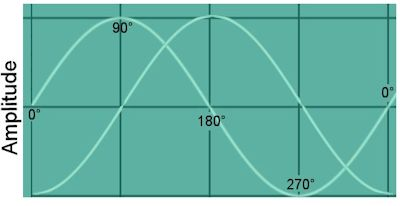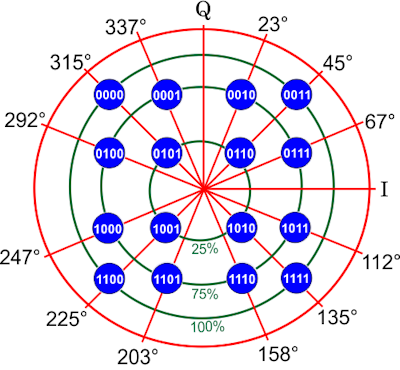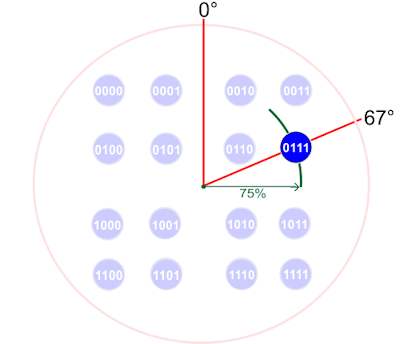# What is QAM and how does it work?

By Michael Spalter
May 2021In my recent article on Wi-Fi 6, I explained that a coding method called QAM was at its heart. QAM is also used in cable modems (DOCSIS), which I'm covering in another article soon.   Explaining QAM is unnecessarily complex to have included in the original Wi-Fi 6 article, but I promised to explain it later and, being such a brilliant and elegant method, it's worth understanding.

What is QAM?To understand the benefits of Wi-Fi 6 and how it provides its benefits doesn't require an understanding of the underlying radio technologies or science. That topic is immensely complex and involves of a lot of maths which is beyond most of us.  One principle however, QAM is particularly interesting and also important as it forms the basis of the signal modulation used in Wi-Fi. That is, how your binary data is actually represented on an analogue waveform.

If you take a basic sine wave. It has a frequency (fixed) and an amplitude (variable). You can press a piano key hard or softly which varies the amplitude but the frequency is always the same (Middle C is 261.6 Hz).  If you plot the wave over time (see right), you can see that it also has 'phase' which cycles smoothly (uniformly at a constant rate), from 0 ° to 360 ° - a wave is therefore 'cyclic'.

An encoding method called BPSK (Binary Phase Shift Keying) takes a wave and by varying the phase, it can encode a simple binary pattern by shifting phase during the cycle between 0 ° and 180 ° to represent a zero or a one. i.e. instead of a smooth SIN wave, it now jumps up and down 'abruptly' which can be used to represent binary data.  By increasing it to four points (0 °, 90 °, 180 ° and 270 °) you can encode 2 bits per symbol (representing 00, 01, 10 or 10); this is called QPSK (Quadrature PSK).Figure 1 : A 16-QAM ConstellationFigure 2 :  '0111' represented by 60 ° @ 75%'

Constellation Diagram

QAM (Quadrature Amplitude Modulation) uses two SIN waves of the same frequency (therefore the same subcarrier) out of phase by 90 ° to each other  (a quarter of the wave, hence the name 'quadrature'). e.g. when one wave is at 0 ° the other is at 90 °, when one is at 10 °, the other is at 100 ° etc.

These two waves are known as the In-phase (I) and Quadrature (Q) signals and they are mathematically combined to make a single wave.  QAM is different to QPSK, however in that an additional factor, amplitude is also varied.  By varying both the phase and the amplitude, more data can be encoded because instead of just an angle offset, the signal can vary in amplitude (how strong it is). The resulting signal is a vector - it has both amplitude and phase.

16-QAM has 16 different possible combinations of amplitude and phase. This is most commonly depicted in a Constellation Diagram (see figure 1, right). Note : Wi-Fi 6 uses 1024-QAM so had 1024 points but we're using 16-QAM for simplicity - the concept is identical.

A constellation diagram depicts visually how each combination of phase and amplitude is mapped to represent a different binary number. As 16-QAM has 16 points, you can therefore represent any number from 0-15 (decimal) or 0000-1111 (binary).   Every point on the diagram is a different distance from the centre origin and at a different angle (phase shift) from 0 °. Figure 2 shows how we would transmit '0111' - you would transmit the tone at 75% amplitude with a 67 ° phase shift (for a duration of 12.8μs, which is the symbol duration in Wi-Fi 6).

You can have any number of points in your QAM constellation - just keep adding dots. Constellations are normally square and as the number of points increases, so does the number of bits that can be encoded, mathematically calculated as Bits=Log2(Points). 16-QAM has 16 points, so a symbol can represent any digit from 0-15 or 0000 to 1111 (4 bits). Wi-Fi 5 uses 256-QAM (8 bits) and Wi-Fi 6 uses 1024-QAM which has 1024 points, representing 0-1023 or 0000000000-1111111111 which is 10 bits so that's there's a 20% capacity increase over Wi-Fi 5.

As you increase the number of QAM points, the points are either closer together or you have to increase power (amplitude) to maintain space between points.  As Wi-Fi systems are limited in power (both by regulation and practicality), using 1024-QAM packs the points closer together.  As you increase point density, the points spread more - there is less separation between points which gives less immunity to noise as the receiver may have difficulty in accurately identifying each point on an imperfect connection. Furthermore, if a signal is weaker, as we're varying amplitude, weaker symbols may not be detectable so a QAM system has to be dynamic and only make use of reliable symbol positions within the constellation.

In Wi-Fi, it's all a lot more complex - there are multiple carriers and complex encoding schemes but the principle of QAM is the same.  QAM isn't just used for WiFi, but many other technologies, including DSL (ADSL, VDSL & G.Fast) and cable-modems (DOCSIS).

Tags

802.11ac
802.11ax
WiFi 6
Wifi 5
OFDMA
QAM
1024-QAM
OFDM
Wi-Fi 6
Wi-Fi 5